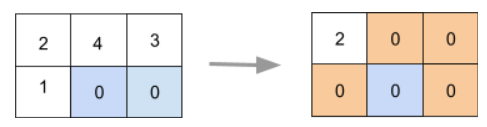# Zero Matrix

Posted: 18 Feb, 2021
Difficulty: Easy

## PROBLEM STATEMENT

You are given a matrix 'MATRIX' of dimension 'N' x 'M'. Your task is to make all the elements of row 'i' and column 'j' equal to 0 if any element in the ith row or jth column of the matrix is 0.

#### Note:

``````1) The number of rows should be at least 1.

2) The number of columns should be at least 1.

3) For example, refer to the below matrix illustration:
``````##### Input Format:
``````The first line of the input contains an integer 'T' denoting the number of test cases.

The first line of each test case contains two space-separated integers, 'N' and 'M', as described in the problem statement.

The next 'N' lines of each test case contain 'M' integers separated by spaces describing rows of the matrix.
``````
##### Output Format:
``````For each test case, return 'N' rows consisting of 'M' integers representing the matrix.
``````
##### Note:
``````You don't need to print anything, it has already been taken care of. Just implement the given function.
``````
##### Constraints:
``````1 <= T <= 50
1 <= N <= 100
1 <= M <= 100
-10^9 <= MATRIX[i][j] <= 10^9

Where 'MATRIX[i][j]' denotes the matrix element.
``````

``````Can you solve it with the space complexity of O(1)?

Time limit: 1 sec
``````Approach 1

We can use two vectors of datatype bool of size ‘N’ and ‘M’, let say ‘ROW’ and 'COL' respectively.

The steps are as follows:

• Initialize both the vectors with the false.
• We are aiming to change the entry of the vector from false to true if we found any element which is 0 in that row for the ‘ROW’ vector and column for the 'COL' vector.
• Run a loop to N times for the ‘ROW’, in each iteration:
• Run a loop to M times for the entries in that ‘ROW’, in each iteration:
• If ‘MATRIX[i][j]' is 0, then mark ‘ROW[i]' = true and ‘COL[j]’ = true because we have to set entire ith row and entire jth column of the matrix ‘MATRIX’ to 0, where 0 <= ‘i’ < ‘N’ and 0 <= ‘j’ < ‘M’ and ‘i’ is for row and ‘j’ is for column.
• After the completion of the above steps, the ‘ROW’ vector contains true for those for which we have to set the entire row 0, and the 'COL' vector contains true for those for which we have to set the entire column 0.
• Again, run a loop to ‘N’ times for the ‘ROW’, in each iteration:
• Run a loop to ‘M’ times for the entries in that ‘ROW’, in each iteration:
• If ‘ROW’[i]' = true or ‘COL[j]’ = true, then set ‘MATRIX[i][j]' = 0.
• Else, leave ‘MATRIX[i][j]' as it is.
• Finally, return the matrix ‘MATRIX’.